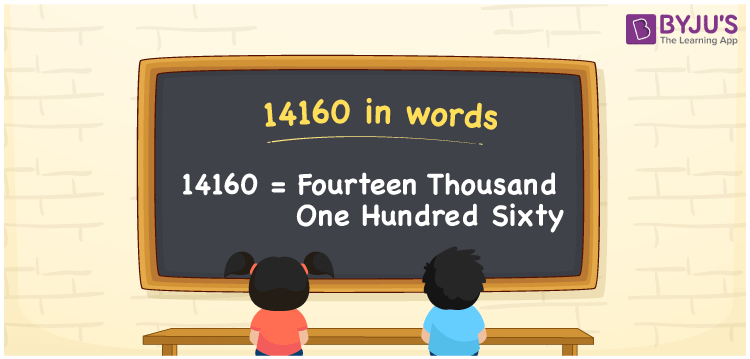# 14160 in words

14160 in words is written as Fourteen Thousand One Hundred and Sixty. In 14160, 1 has a place value of ten thousand and 4 has a place value of thousand, 1 has a place value of hundred and 6 has a place value of tens. The article on Place Value gives more information. The number 14160 is used in expressions that relate to money, distance, length, count and many more. For example, “A book had Fourteen Thousand One Hundred and Sixty pages in it.”

 14160 in words Fourteen Thousand One Hundred and Sixty Fourteen Thousand One Hundred and Sixty in Numbers 14160

## 14160 in English Words## How to Write 14160 in Words?

We can convert 14160 to words using a place value chart. The number 14160 has 5 digits, so let’s make a chart that shows the place value up to 5 digits.

 Ten thousand Thousands Hundreds Tens Ones 1 4 1 6 0

Thus, we can write the expanded form as:

1 × Ten thousand + 4 × Thousand + 1 × Hundred + 6 × Ten + 0 × One

= 1 × 10000 + 4 × 1000 + 1 × 100 + 6 × 10 + 0 × 1

= 14160

= Fourteen Thousand One Hundred and Sixty .

14160 is the natural number that is succeeded by 14159 and preceded by 14161.

14160 in words – Fourteen Thousand One Hundred and Sixty.

Is 14160 an odd number? – No.

Is 14160 an even number? – Yes.

Is 14160 a perfect square number? – No.

Is 14160 a perfect cube number? – No.

Is 14160 a prime number? – No.

Is 14160 a composite number? – Yes.

## Solved Example

1. Write the number 14160 in expanded form

Solution: 1 x 10000 + 4 x 1000 + 1 x 100 + 6 x 10 + 0 x 1

We can write 14160 =10000 + 4000 + 100 + 60 + 0

= 1 x 10000 + 4 x 1000 + 1 x 100 + 6 x 10 + 0 x 1

## Frequently Asked Questions on 14160 in words

Q1

### How to write 14160 in words?

14160 in words is written as Fourteen Thousand One Hundred and Sixty.
Q2

### State whether True or False. 14160 is divisible by 3?

True. 14160 is divisible by 3.
Q3

### Is 14160 divisible by 10?

Yes. 14160 is divisible by 10.